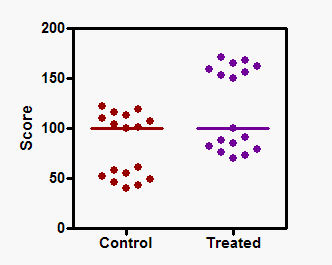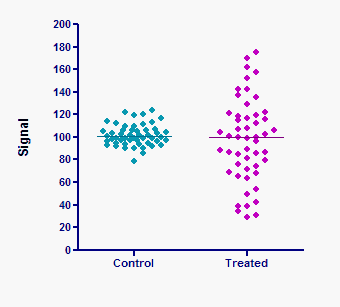KNOWLEDGEBASE - ARTICLE #1327

# The Mann-Whitney test doesn't really compare medians.

You'll sometimes read that the Mann-Whitney test compares the medians of two groups. But this is not always correct (1)

Consider this example:The graph shows each value obtained from control and treated subjects. The two-tail P value from the Mann-Whitney test is 0.0288, so you conclude that there is a statistically significant difference between the groups. But the two medians, shown by the horizontal lines, are identical. The Mann-Whitney test ranked all the values from low to high, and then compared the means  ranks. The mean of the ranks of the control values is much lower than the mean of the ranks of the treated values, so the P value is small, even though the medians of the two groups are identical.

It is also not entirely correct to say that the Mann-Whitney test asks whether the two groups come from populations with different distributions. The two groups in the graph below clearly come from different distributions, but the P value from the Mann-Whitney test is high (0.46). The standard deviation of the two groups is obviously very different. But since the Mann-Whitney test analyzes only the ranks, it does not see a substantial difference between the groups.The Mann-Whitney test compares the mean ranks -- it does not compare medians and does not compare distributions. More generally, the P value answers this question: What is the chance that a randomly selected value from the population with the larger mean rank is greater than than a randomly selected value from the other population?

If you make an additional assumption -- that the distributions of the two populations have the same shape, even if they are shifted (have different medians) -- then the Mann-Whiteny test can be considered a a test of medians. If you accept the assumption of identically shaped distributions, then a small P value from a Mann-Whitney test leads you to conclude that the difference between medians is statistically signficant. But Michael J. Campbell pointed out, "However, if the groups have the same distribution, then a shift in location will move medians and means by the same amount and so the difference in medians is the same as the difference in means. Thus the Mann-Whitney test is also a test for the difference in means."

The Kruskal-Wallis test is the corresponding nonparametric test for comparing three or more groups. Everything on this page about the Mann-Whitney test applies equally to the Kruskal-Wallis test.

1. A. Hart. Mann-Whitney test is not just a test of medians: differences in spread can be important. BMJ (2001) vol. 323 (7309) pp. 391

## Explore the Knowledgebase

Analyze, graph and present your scientific work easily with GraphPad Prism. No coding required.